# JB*-triple

(diff) ← Older revision | Latest revision (diff) | Newer revision → (diff)-triples were introduced by W. Kaup [a8] in connection with the study of bounded symmetric domains in complex Banach spaces. A definition of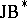-triples involving holomorphy is as follows: A-triple is a complex Banach spacesuch that the open unit ballofis homogeneous under its full groupof biholomorphic automorphisms (and hence is symmetric, cf. Symmetric space). The main result in [a8] states that to every abstract bounded symmetric domainin a complex Banach space there exists a unique (up to linear isometry)-triplewhose open unit ball is biholomorphically equivalent to. The groupis always a real Banach Lie group (cf. also Lie group, Banach) acting in a natural way on various spaces of holomorphic functions as well as on various submanifolds of the unit sphere in(in casehas finite dimension,is semi-simple and also has finite dimension — the induced unitary representation on Bergman space, cf. also Bergman spaces, is of special interest in harmonic analysis).

An equivalent, but more algebraic definition for-triples is as follows: The complex Banach spaceis a-triple if it carries a (necessarily unique) ternary product (called triple product)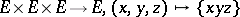satisfying the following properties for all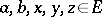and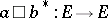defined by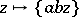:

i)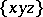is symmetric complex bilinear in the outer variables,and conjugate linear in;

ii)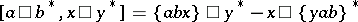(the Jordan triple identity);

iii)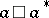, as a linear operator on, is Hermitian and has spectrum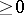(cf. also Hermitian operator; Spectrum of an operator);

iv)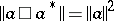(the-condition).

The sesquilinear mappingmay be considered as an operator-valued product on. It satisfies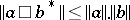(not an elementary fact!) and condition iv) is analogous to the characteristic property of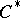-algebras (cf. also-algebra). On the other hand, by iii), the above mapping may also be considered as a positive Hermitian operator-valued form on, thus giving a natural orthogonality relation on.

Some examples are:

1) Every-algebra (more precisely, the underlying complex Banach space). The triple product is given by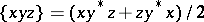.

2) Every closed (complex) subtriple of a-algebra. These are also called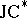-triples. These triples were originally introduced and intensively studied by L.A. Harris [a5] under the name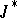-algebra (cf. also Banach–Jordan algebra).

3) Every-algebra (i.e. Jordan-algebra, [a15]), with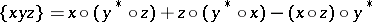. In particular, the famous exceptional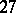-dimensional-algebra(which is not a-triple) of all Hermitian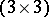-matrices over the complex octonian algebra.

4) Every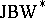-triple, i.e. a-triple having a (necessarily unique) pre-dual. Among these are the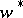-closed subtriples of von Neumann algebras as well as the Cartan factors, which are the building blocks of the-triples of type I (in analogy to the von Neumann algebras of type I, cf. also von Neumann algebra).

The class of all-triples is invariant under taking arbitrary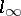-sums, quotients by closed triple ideals, ultrapowers, biduals [a1], as well as contractive projections [a9]. Notice that the range of a contractive projection on a-algebra in general does not have the structure of a-algebra, but always is a-triple. The Gel'fand–Naimark theorem of Y. Friedman and B. Russo [a3] states that each-triple can be realized as a subtriple of an-sumwhereis the-triple of all bounded linear operators on a suitable complex Hilbert space andis the exceptional-algebra of all-valued continuous functions on a suitable compact topological space. By [a6], the classification of-triples can be achieved modulo the classification of von Neumann algebras. Furthermore, in [a11] all prime-triples have been classified using Zel'manov techniques.

The-triples form a large class of complex Banach spaces whose geometry can be described algebraically. Examples of this are:

A bijective linear operator between-triples is an isometry if and only if it respects the Jordan triple product.

The M-ideals inare precisely the closed triple ideals of.

The open unit ball ofis the largest convex subset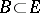containing the origin such that for everythe Bergman operator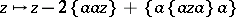is invertible, and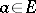is an extreme point of the closed unit ball inif and only if the Bergman operator associated tois the zero operator.

Real-triples were studied in [a7]; these are the real forms of (complex)-triples. In general, a-triple may have many non-isomorphic real forms. An important class of real-triples is obtained from the class of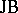-algebras, compare [a4].

How to Cite This Entry:
JB*-triple. Encyclopedia of Mathematics. URL: http://encyclopediaofmath.org/index.php?title=JB*-triple&oldid=18845
This article was adapted from an original article by Wilhelm Kaup (originator), which appeared in Encyclopedia of Mathematics - ISBN 1402006098. See original article